Home Practice
For learners and parents For teachers and schools
Textbooks
Full catalogue
Pricing SupportLog in

We think you are located in United States. Is this correct?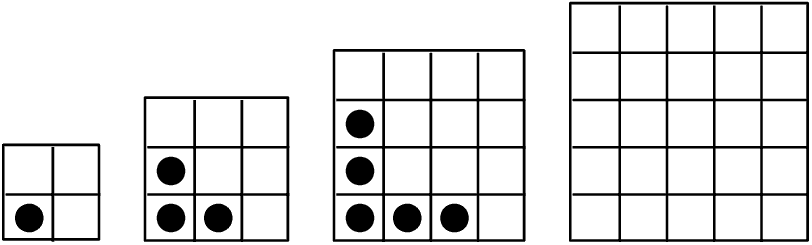1. Study the dotted-tile pattern shown and answer the following questions.
1. Complete the fourth pattern in the diagram.
2. Complete the table below:

 pattern number $$\text{1}$$ $$\text{2}$$ $$\text{3}$$ $$\text{4}$$ $$\text{5}$$ $$\text{20}$$ $$n$$ dotted tiles $$\text{1}$$ $$\text{3}$$ $$\text{5}$$ difference ($$d$$) $$-$$ $$\text{2}$$
3. What do you notice about the change in number of dotted tiles?
4. Describe the pattern in words: “The number of dotted tiles...”.
5. Write the general term: $$T_n = \ldots$$
6. Give the mathematical name for this kind of pattern.
7. A pattern has $$\text{819}$$ dotted tiles. Determine the value of $$n$$.
2. Now study the number of blank tiles (tiles without dots) and answer the following questions:
1. Complete the table below:

 pattern number $$\text{1}$$ $$\text{2}$$ $$\text{3}$$ $$\text{4}$$ $$\text{5}$$ $$\text{10}$$ blank tiles $$\text{3}$$ $$\text{6}$$ $$\text{11}$$ first difference $$-$$ $$\text{3}$$ second difference $$-$$ $$-$$
2. What do you notice about the change in the number of blank tiles?
3. Describe the pattern in words: “The number of blank tiles...”.
4. Write the general term: $$T_n = \ldots$$
5. Give the mathematical name for this kind of pattern.
6. A pattern has $$\text{227}$$ blank tiles. Determine the value of $$n$$.
7. A pattern has $$\text{79}$$ dotted tiles. Determine the number of blank tiles.

A quadratic sequence is a sequence of numbers in which the second difference between any two consecutive terms is constant.

Consider the following example: $$1; 2; 4; 7; 11; \ldots$$

The first difference is calculated by finding the difference between consecutive terms:The second difference is obtained by taking the difference between consecutive first differences:We notice that the second differences are all equal to $$\text{1}$$. Any sequence that has a common second difference is a quadratic sequence.

It is important to note that the first differences of a quadratic sequence form a sequence. This sequence has a constant difference between consecutive terms. In other words, a linear sequence results from taking the first differences of a quadratic sequence.

temp text

General case

If the sequence is quadratic, the $$n^{\text{th}}$$ term is of the form $${T}_{n}=a{n}^{2}+bn+c$$.In each case, the common second difference is a $$2a$$.

Textbook Exercise 3.2

Determine the second difference between the terms for the following sequences:

$$5; 20; 45; 80; \ldots$$

\begin{align*} \text{First differences: } &= 15; 25; 35 \\ \text{Second difference: } &= 10 \end{align*}

$$6; 11; 18; 27; \ldots$$

\begin{align*} \text{First differences: } &= 5; 7; 9 \\ \text{Second difference: } &= 2 \end{align*}

$$1; 4; 9; 16; \ldots$$

\begin{align*} \text{First differences: } &= 3; 5; 7 \\ \text{Second difference: } &= 2 \end{align*}

$$3; 0; -5; -12; \ldots$$

\begin{align*} \text{First differences: } &= -3; -5; -7 \\ \text{Second difference: } &= -2 \end{align*}

$$1; 3; 7; 13; \ldots$$

\begin{align*} \text{First differences: } &= 2; 4; 6 \\ \text{Second difference: } &= 2 \end{align*}

$$0; -6; -16; -30; \ldots$$

\begin{align*} \text{First differences: } &=-6; -10; -14 \\ \text{Second difference: } &= -4 \end{align*}

$$-1; 2; 9; 20; \ldots$$

\begin{align*} \text{First differences: } &= 3; 7; 11 \\ \text{Second difference: } &= 4 \end{align*}

$$1; -3; -9; -17; \ldots$$

\begin{align*} \text{First differences: } &= -4; -6; -8 \\ \text{Second difference: } &= -2 \end{align*}

$$3a+1; 12a+1; 27a+1; 48a+1 \ldots$$

\begin{align*} \text{First differences: } &= 9a; 15a; 21a \\ \text{Second difference: } &= 6a \end{align*}

$$2; 10; 24; 44; \ldots$$

\begin{align*} \text{First differences: } &= 8; 14; 20 \\ \text{Second difference: } &= 6 \end{align*}

$$t-2; 4t-1; 9t; 16t+1; \ldots$$

\begin{align*} \text{First differences: } &= 3t + 1; 5t +1; 7t + 1 \\ \text{Second difference: } &= 2t \end{align*}

Complete the sequence by filling in the missing term:

$$11; 21; 35; \ldots; 75$$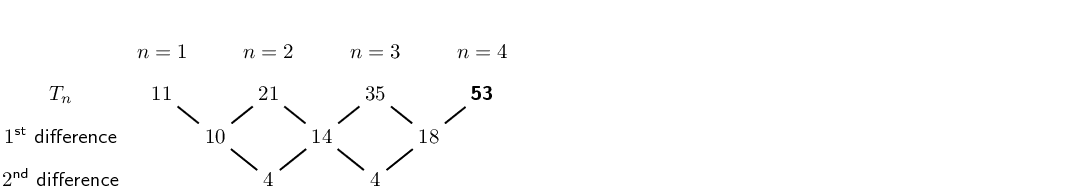$$20; \ldots; 42; 56; 72$$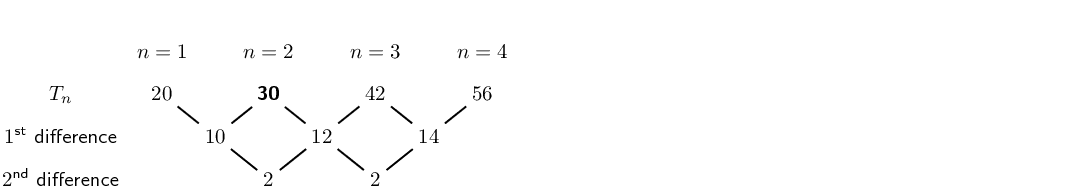$$\ldots; 37; 65; 101$$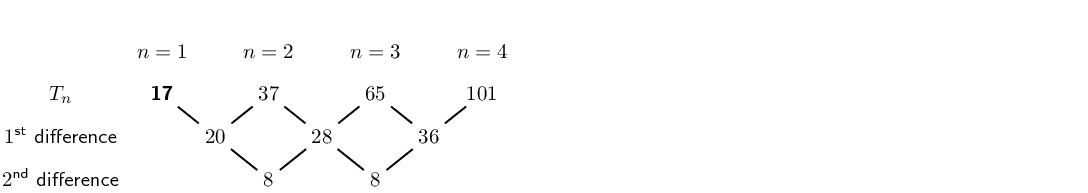$$3; \ldots; -13; -27; -45$$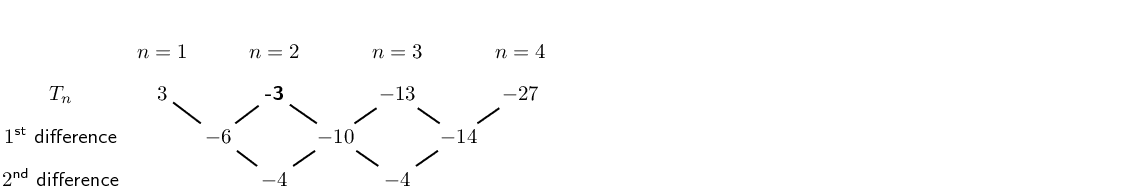$$24; 35; 48; \ldots; 80$$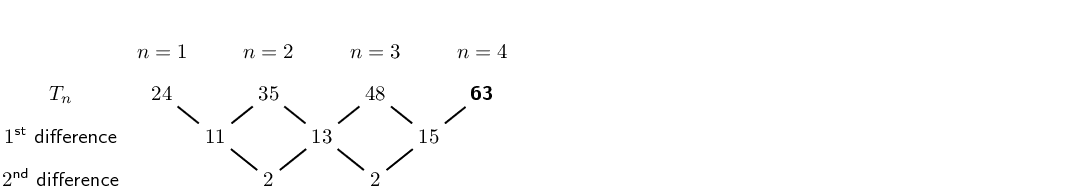$$\ldots; 11; 26; 47$$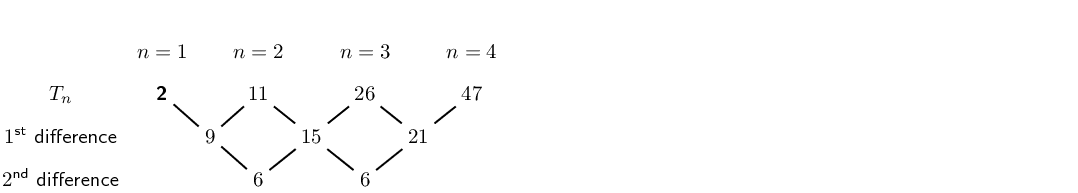Use the general term to generate the first four terms in each sequence:

$$T_n = n^2+3n-1$$

$$3;9;17;27$$

$$T_n = -n^2-5$$

$$-6;-9;-14;-21$$

$$T_n = 3n^2-2n$$

$$1;8;21;40$$

$$T_n = -2n^2+n+1$$

$$0;-5;-14;-27$$

## Worked example 2: Quadratic sequences

Write down the next two terms and determine an equation for the $$n^{\text{th}}$$ term of the sequence $$\text{5}$$; $$\text{12}$$; $$\text{23}$$; $$\text{38}$$; $$\ldots$$

### Find the first differences between the terms### Find the second differences between the termsSo there is a common second difference of $$\text{4}$$. We can therefore conclude that this is a quadratic sequence of the form $$T_n = an^2 + bn + c$$.

Continuing the sequence, the next first differences will be:### Finding the next two terms in the sequence

The next two terms will be:So the sequence will be: $$\text{5}$$; $$\text{12}$$; $$\text{23}$$; $$\text{38}$$; $$\text{57}$$; $$\text{80}$$; $$\ldots$$

### Determine the general term for the sequence

To find the values of $$a$$, $$b$$ and $$c$$ for $$T_n = an^2 + bn + c$$ we look at the first $$\text{3}$$ terms in the sequence:

\begin{align*} n=1: T_1 &= a + b + c \\ n=2: T_2 &= 4a + 2b + c \\ n=3: T_3 &= 9a + 3b + c \end{align*}

We solve a set of simultaneous equations to determine the values of $$a$$, $$b$$ and $$c$$

We know that $$T_1 = 5$$, $$T_2 = 12$$ and $$T_3 = 23$$

\begin{align*} a + b + c &= 5 \\ 4a + 2b + c &= 12 \\ 9a + 3b + c &= 23 \end{align*}\begin{align*} T_2 - T_1 &= 4a + 2b + c - (a + b + c) \\ 12 - 5 &= 4a + 2b + c - a - b - c \\ 7 &= 3a + b \qquad \ldots (1) \end{align*}\begin{align*} T_3 - T_2 &= 9a + 3b + c - (4a + 2b + c) \\ 23 - 12 &= 9a + 3b + c - 4a - 2b - c \\ 11 &= 5a + b \qquad \ldots (2) \end{align*}\begin{align*} (2)-(1) &= 5a + b - (3a + b) \\ 11 - 7 &= 5a + b - 3a - b \\ 4 &= 2a \\ \therefore a &= 2 \end{align*}\begin{align*} \text{Using equation } (1): \quad 3(2) + b &= 7 \\ \therefore b &= 1 \\ \text{And using } \quad a + b + c &= 5 \\ 2 + 1 + c &= 5 \\ \therefore c &= 1 \end{align*}

### Write the general term for the sequence

$T_n = 2n^2 + n + 2$

temp text

## Worked example 3: Plotting a graph of terms in a sequence

Consider the following sequence:

$3; 6; 10; 15; 21; \ldots$

1. Determine the general term ($$T_n$$) for the sequence.
2. Is this a linear or a quadratic sequence?
3. Plot a graph of $$T_n$$ vs $$n$$.

### Determine the first and second differences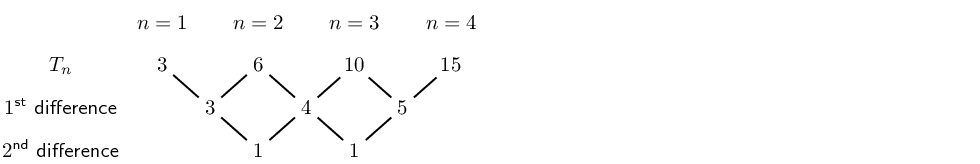We see that the first differences are not constant and form the sequence $${3; 4; 5; \ldots}$$ and that there is a common second difference of $$\text{1}$$. Therefore the sequence is quadratic and has a general term of the form $$T_n = an^2 + bn + c$$.

### Determine the general term $$T_n$$

To find the values of $$a$$, $$b$$ and $$c$$ for $$T_n = an^2 + bn + c$$ we look at the first $$\text{3}$$ terms in the sequence:

\begin{align*} n=1: T_1 &= a + b + c \\ n=2: T_2 &= 4a + 2b + c \\ n=3: T_3 &= 9a + 3b + c \end{align*}

We solve this set of simultaneous equations to determine the values of $$a$$, $$b$$ and $$c$$. We know that $$T_1 = 3$$, $$T_2 = 6$$ and $$T_3 = 10$$.

\begin{align*} a + b + c &= 3 \\ 4a + 2b + c &= 6 \\ 9a + 3b + c &= 10 \end{align*}\begin{align*} T_2 - T_1 &= 4a + 2b + c - (a + b + c) \\ 6 - 3 &= 4a + 2b + c - a - b - c \\ 3 &= 3a + b \qquad \ldots (1) \end{align*}\begin{align*} T_3 - T_2 &= 9a + 3b + c - (4a + 2b + c) \\ 10 - 6 &= 9a + 3b + c - 4a - 2b - c \\ 4 &= 5a + b \qquad \ldots (2) \end{align*}\begin{align*} (2)-(1) &= 5a + b - (3a + b) \\ 4 - 3 &= 5a + b - 3a - b \\ 1 &= 2a \\ \therefore a &= \frac{1}{2} \end{align*}\begin{align*} \text{Using equation }(1): \quad 3\left(\frac{1}{2}\right) + b &= 3 \\ \therefore b &= \frac{3}{2} \\ \text{And using } \quad a + b + c &= 3 \\ \frac{1}{2} + \frac{3}{2} + c &= 3 \\ \therefore c &= 1 \end{align*}

Therefore the general term for the sequence is $$T_n = \frac{1}{2}n^2 + \frac{3}{2}n + 1$$.

### Plot a graph of $$T_n$$ vs $$n$$

Use the general term for the sequence, $$T_n = \frac{1}{2}n^2 + \frac{3}{2}n + 1$$, to complete the table.

 $$n$$ $$\text{1}$$ $$\text{2}$$ $$\text{3}$$ $$\text{4}$$ $$\text{5}$$ $$\text{6}$$ $$\text{7}$$ $$\text{8}$$ $$\text{9}$$ $$\text{10}$$ $$T_n$$ $$\text{3}$$ $$\text{6}$$ $$\text{10}$$ $$\text{15}$$ $$\text{21}$$ $$\text{28}$$ $$\text{36}$$ $$\text{45}$$ $$\text{55}$$ $$\text{66}$$

Use the table to plot the graph: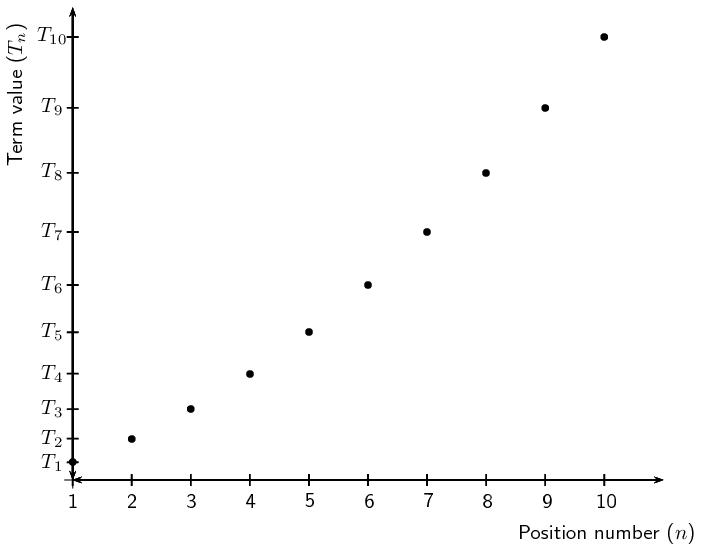In this case it would not be accurate to join these points, since $$n$$ indicates the position of a term in a sequence and can therefore only be a positive integer. We can, however, see that the plot of the points lies in the shape of a parabola.

temp text

## Worked example 4: Olympic Games soccer event

In the first stage of the soccer event at the Olympic Games, there are teams from four different countries in each group. Each country in a group must play every other country in the group once.

1. How many matches will be played in each group in the first stage of the event?
2. How many matches would be played if there are $$\text{5}$$ teams in each group?
3. How many matches would be played if there are $$\text{6}$$ teams in each group?
4. Determine the general formula of the sequence.

### Determine the number of matches played if there are $$\text{4}$$ teams in a group

Let the teams from four different countries be $$A$$, $$B$$, $$C$$ and $$D$$.

 teams in a group matches played $$A$$ $$AB, AC, AD$$ $$B$$ $$BC, BD$$ $$C$$ $$CD$$ $$D$$ $$\text{4}$$ $$3+2+1=6$$

$$AB$$ means that team $$A$$ plays team $$B$$ and $$BA$$ would be the same match as $$AB$$. So if there are four different teams in a group, each group plays $$\text{6}$$ matches.

### Determine the number of matches played if there are $$\text{5}$$ teams in a group

Let the teams from five different countries be $$A, B, C, D$$ and $$E$$.

 teams in a group matches played $$A$$ $$AB, AC, AD, AE$$ $$B$$ $$BC, BD, BE$$ $$C$$ $$CD, CE$$ $$D$$ $$DE$$ $$E$$ $$\text{5}$$ $$4+3+2+1=10$$

So if there are five different teams in a group, each group plays $$\text{10}$$ matches.

### Determine the number of matches played if there are $$\text{6}$$ teams in a group

Let the teams from six different countries be $$A, B, C, D, E$$ and $$F$$.

 teams in a group matches to be played $$A$$ $$AB, AC, AD, AE, AF$$ $$B$$ $$BC, BD, BE, BF$$ $$C$$ $$CD, CE, CF$$ $$D$$ $$DE, DF$$ $$E$$ $$EF$$ $$F$$ $$\text{5}$$ $$5+4+3+2+1=15$$

So if there are six different teams in a group, each group plays $$\text{15}$$ matches.

We continue to increase the number of teams in a group and find that a group of $$\text{7}$$ teams plays $$\text{21}$$ matches and a group of $$\text{8}$$ teams plays $$\text{28}$$ matches.

### Consider the sequence

We examine the sequence to determine if it is linear or quadratic:We see that the first differences are not constant and that there is a common second difference of $$\text{1}$$. Therefore the sequence is quadratic and has a general term of the form $$T_n = an^2 + bn + c$$.

### Determine the general term $$T_n$$

To find the values of $$a$$, $$b$$ and $$c$$ for $$T_n = an^2 + bn + c$$ we look at the first $$\text{3}$$ terms in the sequence:

\begin{align*} n=1: T_1 &= a + b + c \\ n=2: T_2 &= 4a + 2b + c \\ n=3: T_3 &= 9a + 3b + c \end{align*}

We solve a set of simultaneous equations to determine the values of $$a$$, $$b$$ and $$c$$. We know that $$T_1 = 6$$, $$T_2 = 10$$ and $$T_3 = 15$$

\begin{align*} a + b + c &= 6 \\ 4a + 2b + c &= 10 \\ 9a + 3b + c &= 15 \end{align*}\begin{align*} T_2 - T_1 &= 4a + 2b + c - (a + b + c) \\ 10 - 6 &= 4a + 2b + c - a - b - c \\ 4 &= 3a + b \qquad \ldots (1) \end{align*}\begin{align*} T_3 - T_2 &= 9a + 3b + c - (4a + 2b + c) \\ 15- 10 &= 9a + 3b + c - 4a - 2b - c \\ 5 &= 5a + b \qquad \ldots (2) \end{align*}\begin{align*} (2)-(1) &= 5a + b - (3a + b) \\ 5-4 &= 5a + b - 3a - b \\ 1 &= 2a \\ \therefore a &= \frac{1}{2} \end{align*}\begin{align*} \text{Using equation } (1): \quad 3\left(\frac{1}{2}\right) + b &= 4 \\ \therefore b &= \frac{5}{2} \\ \text{And using } a + b + c &= 6 \\ \frac{1}{2} + \frac{5}{2} + c &= 6 \\ \therefore c &= 3 \end{align*}

Therefore the general term for the sequence is $$T_n = \frac{1}{2}n^2 + \frac{5}{2}n + 3$$.

temp text

Textbook Exercise 3.3

Calculate the common second difference for each of the following quadratic sequences:

$$3; \; 6; \; 10; \; 21; \; ...$$

\begin{align*} \text{First differences: } &= 3;4;5;6 \\ \text{Second difference: } &= 1 \end{align*}

$$4; \; 9; \; 16; \; 25; \; ...$$

\begin{align*} \text{First differences: } &= 5;7;9;11 \\ \text{Second difference: } &= 2 \end{align*}

$$7; \; 17; \; 31; \; 49; \; ...$$

\begin{align*} \text{First differences: } &= 10;14;18;22 \\ \text{Second difference: } &= 4 \end{align*}

$$2; \; 10; \; 26; \; 50; \; 82 ...$$

\begin{align*} \text{First differences: } &= 8;16;24;32 \\ \text{Second difference: } &= 8 \end{align*}

$$31; \; 30; \; 27; \; 22; \; 15; \; ...$$

\begin{align*} \text{First differences: } &= -1;-3;-5;-7 \\ \text{Second difference: } &= -2 \end{align*}

Find the first five terms of the quadratic sequence defined by: $$T_n = 5n^2 + 3n + 4$$.

\begin{align*} T_n &= 5n^2 + 3n + 4 \\ T_1 &= 5(1)^2 + 3(1) + 4 \\ &= 12 \\ T_2 &= 5(2)^2 + 3(2) + 4 \\ &= 30 \\ T_3 &= 5(3)^2 + 3(3) + 4 \\ &= 58 \\ T_4 &= 5(4)^2 + 3(4) + 4 \\ &= 96 \\ T_5 &= 5(5)^2 + 3(5) + 4 \\ &= 144 \end{align*} $$12;30;58;96;144$$

Given $$T_n = 4n^2 + 5n + 10$$, find $$T_9$$.

\begin{align*} T_n &= 4n^2 + 5n + 10 \\ T_9 &= 4(9)^2 + 5(9) + 10 \\ &= 379 \end{align*}

Given $$T_n = 2n^2$$, for which value of $$n$$ does $$T_n = 32$$?

\begin{align*} T_n &= 2n^2 \\ 32 &= 2n^2 \\ 16 &= n^2 \\ 4 &= n \end{align*}

Write down the next two terms of the quadratic sequence: $$16; 27; 42; 61; \ldots$$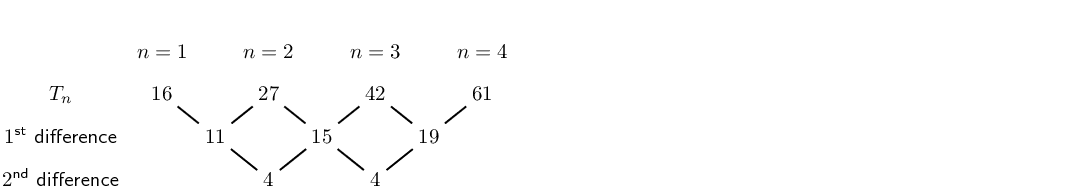Find the general formula for the quadratic sequence above.

\begin{align*} T_n &= an^2 +bn +c \\ T_1 &= a +b +c \\ T_2 &= 4n^2 +2n +c \\ T_3 &= 9n^2 +3n +c \end{align*} \begin{align*} \therefore a + b+ c &= 16 \\ \therefore c &= 16 - a - b \\ 4a + 2b + c &= 27 \\ 4a + 2b + (16 - a - b) &= 27 \\ 3a + b &= 11 \\ \text{And } 9a + 3b + c &= 42 \\ \therefore 9a + 3b + (16 - a - b) &= 42 \\ 8a + 2b = 26 \\ 4a + b = 13 \\ \therefore b &= 13 -4a \end{align*} \begin{align*} \therefore 3a + b &= 11 \\ 3a + (13 - 4a) &= 11 \\ -a &= -2 \\ \therefore a &= 2 \\ b &= 13 -4(2) \\ \therefore b &= 5 \\ \text{And } c &= 16 - a - b \\ \therefore c &= 16 - 2 -5 \\ &= 9 \\ \therefore T_n &= 2n^2 + 5n + 9 \end{align*}